# Simple Harmonic Motion Frequency

The frequency of simple harmonic motion like a mass on a spring is determined by the mass m and the stiffness of the spring expressed in terms of a spring constant k ( see Hooke's Law):If the period is T =s
then the frequency is f = Hz and the angular frequency = rad/s.

The motion is described by

Angular Frequency = sqrt (Spring constant / (Mass )

 ω = sqrt (k / m )
 rad/s = sqrt ( N/m / kg )

Any of the parameters in the equation can be calculated by clicking on the active word in the relationship above. Default values will be entered for any missing data, but those values may be changed and the calculation repeated.

 Resonant frequency expressions Motion equations Motion calculation Motion sequence visualization
Index

Periodic motion concepts

 HyperPhysics***** Mechanics R Nave
Go Back

# Mass on Spring Resonance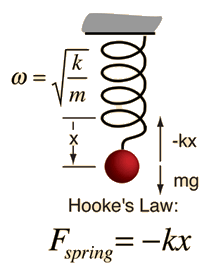A mass on a spring has a single resonant frequency determined by its spring constant k and the mass m. Using Hooke's law and neglecting damping and the mass of the spring, Newton's second law gives the equation of motion: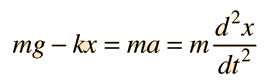The solution to this differential equation is of the form: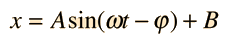which when substituted into the motion equation gives:Collecting terms gives B=mg/k, which is just the stretch of the spring by the weight, and the expression for the resonant vibrational frequency: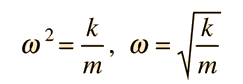This kind of motion is called simple harmonic motion and the system a simple harmonic oscillator.
 Motion equations Motion calculation Frequency calculation Motion sequence visualization
Index

Periodic motion concepts

 HyperPhysics***** Mechanics R Nave
Go Back

# Mass on Spring: Motion Sequence

A mass on a spring will trace out a sinusoidal pattern as a function of time, as will any object vibrating in simple harmonic motion. One way to visualize this pattern is to walk in a straight line at constant speed while carriying the vibrating mass. Then the mass will trace out a sinusoidal path in space as well as time.### Show energy transformation involved in this motion

Index

Periodic motion concepts

 HyperPhysics***** Mechanics R Nave
Go Back

# Energy in Mass on Spring

The simple harmonic motion of a mass on a spring is an example of an energy transformation between potential energy and kinetic energy. In the example below, it is assumed that 2 joules of work has been done to set the mass in motion.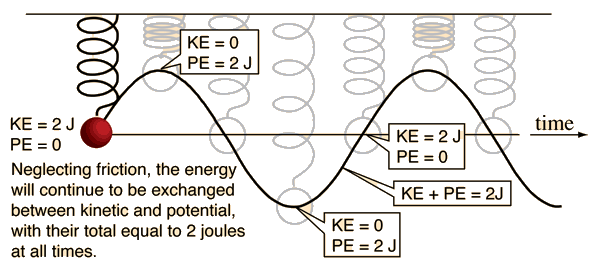Discussion of oscillation energy
Index

Periodic motion concepts

 HyperPhysics***** Mechanics R Nave
Go Back